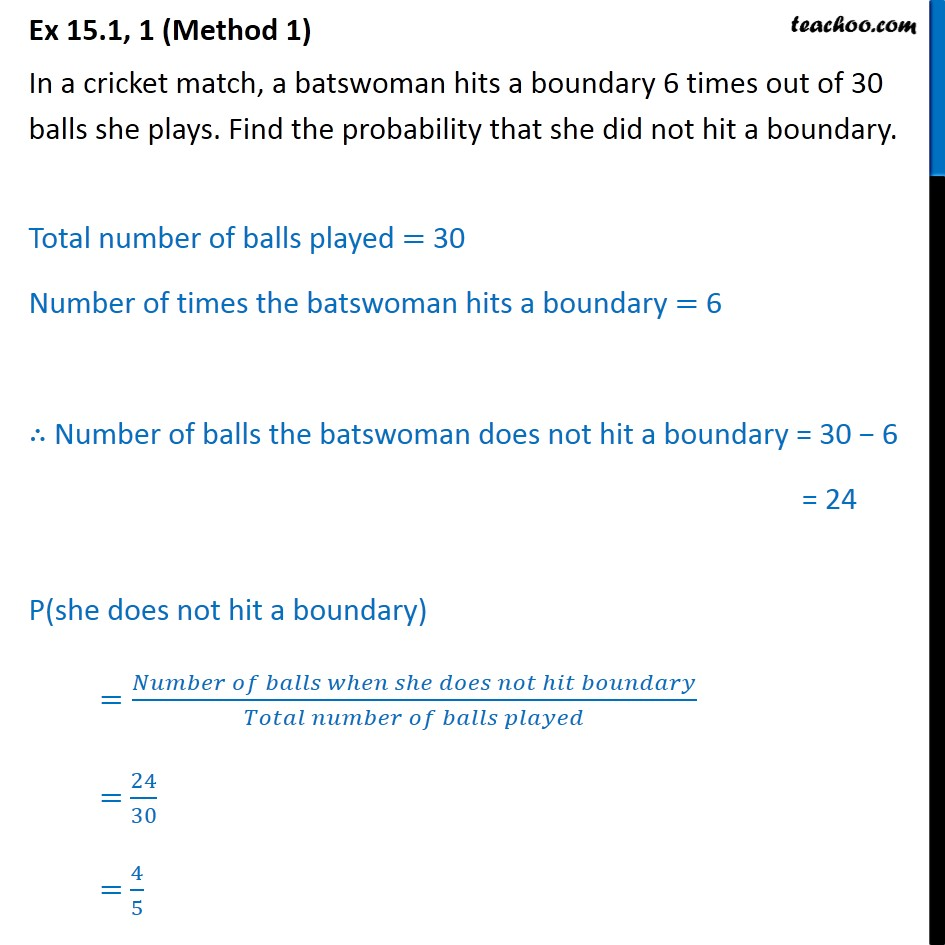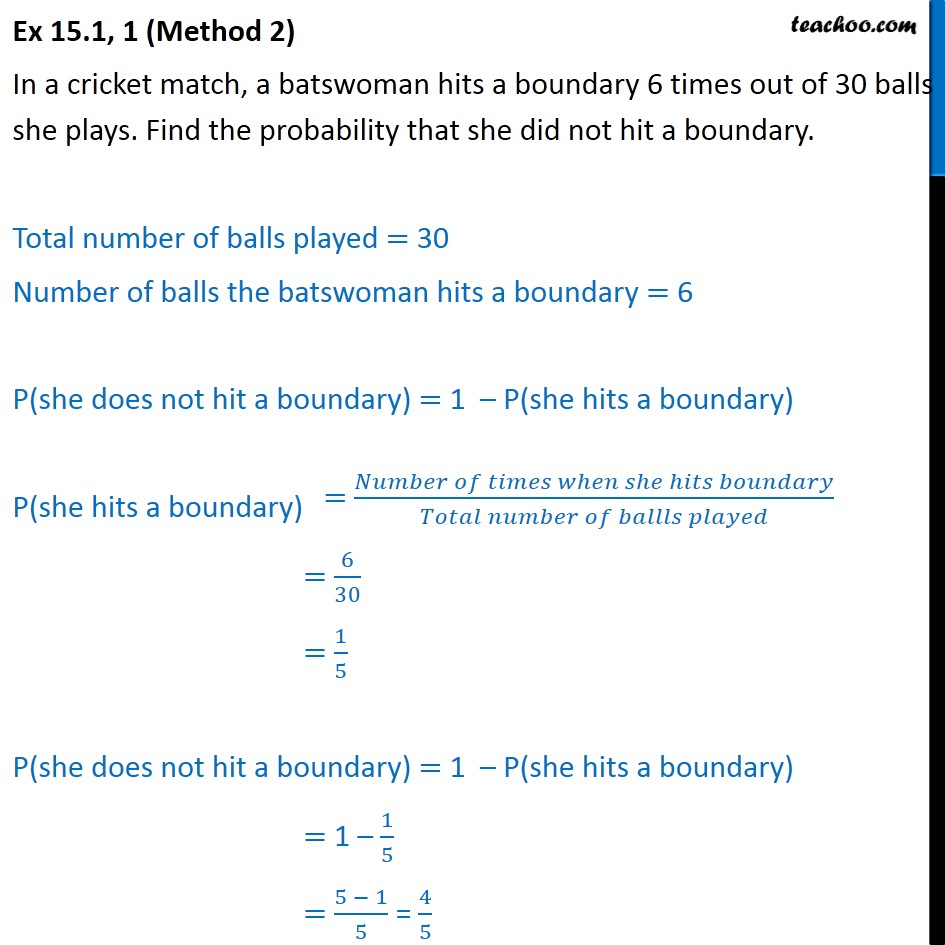Questions - Basic Probability

Probability
Serial order wise
• Questions - Basic Probability

• ExamplesLearn in your speed, with individual attention - Teachoo Maths 1-on-1 Class

### Transcript

Question 1 (Method 1) In a cricket match, a batswoman hits a boundary 6 times out of 30 balls she plays. Find the probability that she did not hit a boundary. Total number of balls played = 30 Number of times the batswoman hits a boundary = 6 Number of balls the batswoman does not hit a boundary = 30 6 = 24 P(she does not hit a boundary) =( )/( ) = 24/30 = 4/5 Question 1 (Method 2) In a cricket match, a batswoman hits a boundary 6 times out of 30 balls she plays. Find the probability that she did not hit a boundary. Total number of balls played = 30 Number of balls the batswoman hits a boundary = 6 P(she does not hit a boundary) = 1 P(she hits a boundary) P(she hits a boundary) = 6/30 = 1/5 P(she does not hit a boundary) = 1 P(she hits a boundary) = 1 1/5 = (5 1)/5 = 4/5